Get instant live expert help with Excel or Google Sheets“My Excelchat expert helped me in less than 20 minutes, saving me what would have been 5 hours of work!”

#### Post your problem and you’ll get expert help in seconds.

Your message must be at least 40 characters
Our professional experts are available now. Your privacy is guaranteed.

# How to Set Up a Random Team Generator in Excel

Excel allows us to create a random team generator using the RAND, RANK and CEILING functions. This step by step tutorial will assist all levels of Excel users to learn how to set up a random team generator in Excel.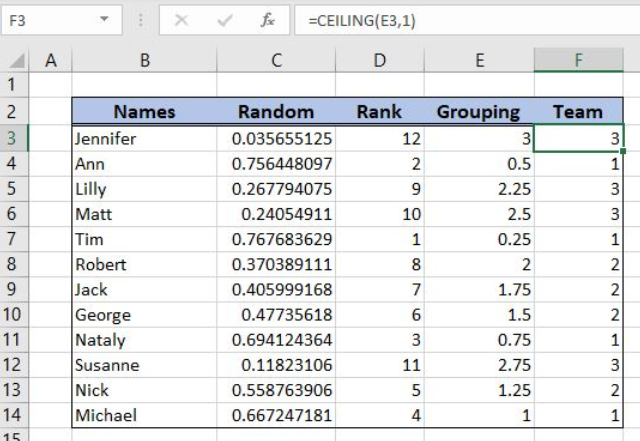Figure 1. The final result of the formula

## Syntax of the RAND Formula

The generic formula for the RAND function is:

`=RAND()`

The function returns a random decimal number between 0 and 1 and has no parameters.

## Syntax of the RANK Formula

The generic formula for the RANK function is:

`=RANK(number, ref, [order])`

The parameters of the RANK function are:

• number – a number which we want to rank
• ref – a list of numbers for ranking
• [order] – 1 for ascending or 0 for descending order. This parameter is optional, if it’s omitted, the default order is descending.

## Syntax of the CEILING Formula

The generic formula for the CEILING function is:

`=CEILING(number, significance)`

The parameters of the RANK function are:

• number – a number which we want to round up to the nearest significance
• significance – a significance for rounding a number up.

## Setting up Our Data for the Formula

Let’s look at the data that we will use in the example. To group names into teams, we will need to have several helper columns. In column B (“Names”), we have names of team members. In column C (“Random”), we will have a random number 0-1 for every name. In column D (“Rank”), we will get a rank for every random number. In column E (“Grouping”), we’ll divide the rank with 3, as we have 3 teams. Finally, in column F (“Team”), we’ll get the team (1, 2 or 3) for each name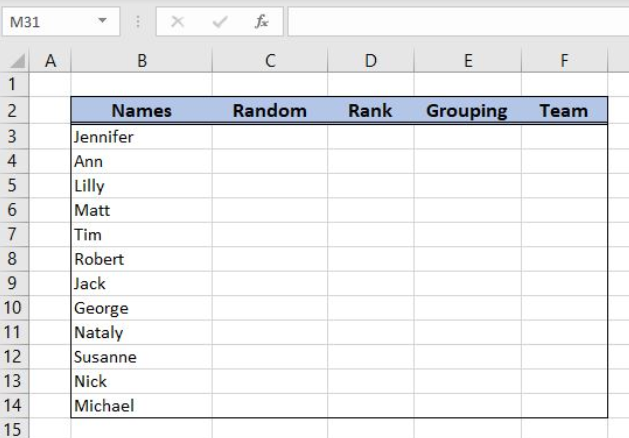Figure 2. The data structure that we will use in the example

## Set up a Random Team Generator

First, we need to get a random number in column C for each name.

The formula for RAND in C3 looks like:

`=RAND()`

To apply the formula, we need to follow these steps:

• Select cell C3 and click on it
• Insert the formula: `=RAND()`
• Press enter
• Drag the formula down to the other cells in the column by clicking and dragging the little “+” icon at the bottom-right of the cell.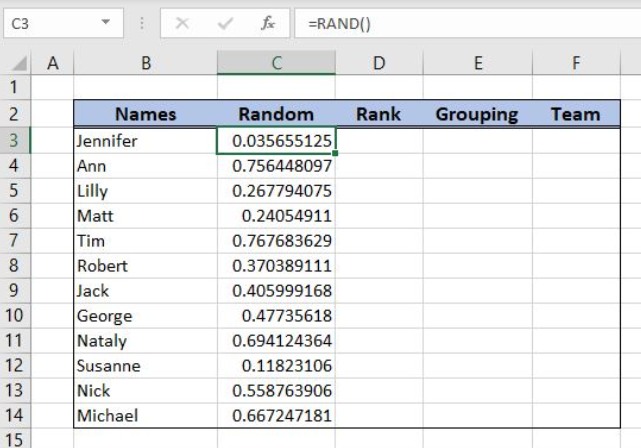Figure 3. Using the RAND formula

When we get a random number for each name we can rank them in column D.

The formula for RANK in C3 looks like:

`=RANK(C3, \$C\$3:\$C\$14)`

The number parameter is the cell C3. The ref parameter is the range \$C\$3:\$C\$14. We must fix the range, as it’s not changing when the formula is copied down the cells.

To apply the formula, we need to follow these steps:

• Select cell D3 and click on it
• Insert the formula: `=RANK(C3,\$C\$3:\$C\$14)`
• Press enter
• Drag the formula down to the other cells in the column by clicking and dragging the little “+” icon at the bottom-right of the cell.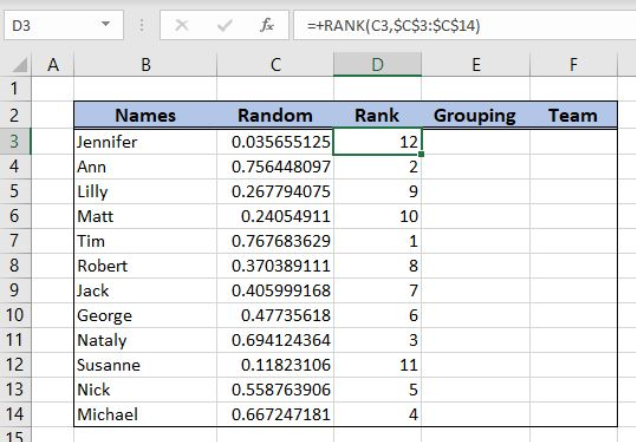Figure 4. Using the RANK formula

Now we have the rank for every random number in column D and can divide them by 3.

The formula in E3 looks like:

`=RANK/D3`

To apply the formula, we need to follow these steps:

• Select cell E3 and click on it
• Insert the formula: `=RANK/D3`
• Press enter
• Drag the formula down to the other cells in the column by clicking and dragging the little “+” icon at the bottom-right of the cell.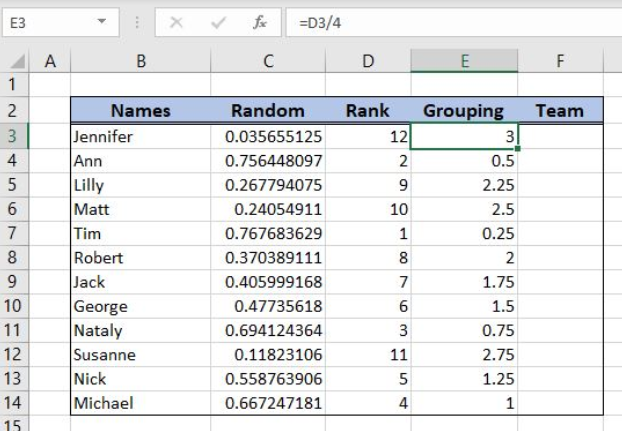Figure 5. Using the formula for grouping

Finally, we can use the CEILING formula, to assign team 1, 2 or 3 to each name.

The formula for CEILING in F3 looks like:

`=CEILING(E3, 1)`

The parameter number is the cell E3, while the significance is 1.

To apply the formula, we need to follow these steps:

• Select cell F3 and click on it
• Insert the formula: `=CEILING(E3,1)`
• Press enter
• Drag the formula down to the other cells in the column by clicking and dragging the little “+” icon at the bottom-right of the cell.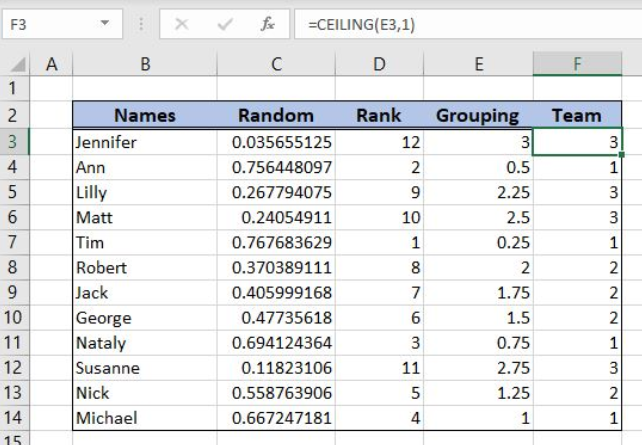Figure 6. Using the CEILING formula

As you can see in Figure 6, in “Team” column we have assigned a team for each name from column B.

Most of the time, the problem you will need to solve will be more complex than a simple application of a formula or function. If you want to save hours of research and frustration, try our live Excelchat service! Our Excel Experts are available 24/7 to answer any Excel question you may have. We guarantee a connection within 30 seconds and a customized solution within 20 minutes.

Solution examplesim creating a fantasy hockey model and trying to figure out how to rank if someone has played more than a certain number of games, and if they're certain position how to rank them accordingly
Solved by X. D. in 60 minsI'm Apply a =RANDBETWEEN formula in one cells want to apply Formula to entire column and run formula when i hit the entire on the particular cell
Solved by M. S. in 20 minsHelp! In a coin toss I have a 1 in 2 (fifty-fifty) chance of getting heads or tails. I need a formula to determine my chance of getting 4 heads in a row or 4 tails in a row out of 10 coin tosses. If I get 4 in a row I win. What formula can I use to determine my chances of winning if I repeat the 10 tosses for hundreds or thousands of times? I get as far as =RANDBETWEEN(0,1) but I have not been able to figure out what formula to use to determine if there are 4 in a row in a group of 10 tosses, times thousands of groups with 10 tosses in each group. Thank-you for any help you can provide.
Solved by V. F. in 51 minsI have 4 column. when i fill the first 3 column. in 4th column i need a unique id number and i generate unique id number using RANDBETWEEN formula [ =CHAR(RANDBETWEEN(65,90))&CHAR(RANDBETWEEN(65,90))&RANDBETWEEN(1000,9900) ] but i want ..... when i'm filling the 3 column after that 4th column run this formula and generate unique id automatically
Solved by X. Q. in 20 minsI cannot enter this formula into Excel. I get an error message asking for an apostrophe?IF(\$S2=0,"",IF(\$S2>0,RANK(\$S2,\$S\$2:\$S\$8000),RANK(\$S2,\$S\$2:\$S\$8000-COUNTIF(\$S\$2:\$S\$8000,0)))
Solved by B. W. in 20 mins